## Example Questions

### Example Question #228 : Integers

My sister invited me to play an online word game. In the game vowels (a,e,i,o,u) are worth 3 points and consonants are worth 5. How much would I score if I use the word “University” ?

Possible Answers:

45

63

42

30

Correct answer:

42

Explanation:

In the word we have 4 vowels (3 x 4 = 12 points) and 6 consonants (5 x 6= 30). If we add the points together we get a total of 42 points.

### Example Question #229 : Integers

What is the sum of multiples of 10 from 10 to 140 inclusive?

Possible Answers:
1050
1000
900
1100
950
Correct answer: 1050
Explanation:

Listing them all, 10-20-30-40-50-60-70-80-90-100-110-120-130-140 you see you can divide the numbers in half (7 pairs). Alternatively you can take (140-10+10)/2/10, adding that additional +10 in the numerator because it is inclusive, giving you 7. Just adding the top and bottom numbers gives you 10+140 for 150. 150*7 is 1050.

### Example Question #230 : Integers

The sum of three consecutive even numbers is 42. What is the smallest even number in this sequence?

Possible Answers:Correct answer:Explanation:

To solve this problem. First set up a mathematical equation that represents this scenario.

"The sum of three consecutive even numbers is 42."

Let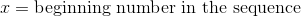and recalling that each consecutive even number is two values greater that the previous even number, the mathematical equation becomes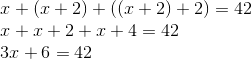From here isolate the variable on one side of the equations with all other constants on the other side.

Subtract six from both sides.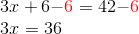Now divide by three.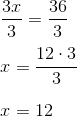Sincerepresents the beginning number in this sequence it also represents the smallest even number in this sequence. Therefore the smallest even number is 12.

### Example Question #871 : Arithmetic

Examine the sequence: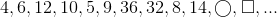Give the number that replaces the square.

Possible Answers: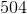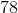Correct answer:Explanation:

After the first term, the next four terms are obtained by adding, multiplying, subtracting, and dividing by 2, in order. The four terms after that are obtained by carrying out the same operations with 4. The next term is obtained by adding 6, so the operations can be expected to be repeated with 6, the next even number.

Observe the operations as carried out with 2: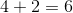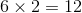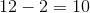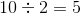Carrying out the same steps, in order, with 4: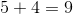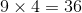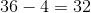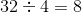Carrying out the same steps, in order, with 6: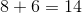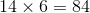, the number that replaces the circle;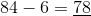, the number that replaces the square.

### Example Question #872 : Arithmetic

Find the values of the variablesandin the following sequence: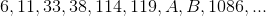Possible Answers: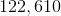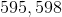None of these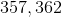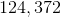Correct answer:Explanation:

Terms are obtained by alternately adding 5 and multiplying by 3: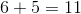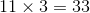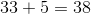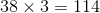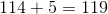The missing two elements are found by multiplying by 3, then by adding 5: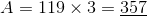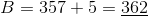Multiplying by 3 confirms that this is correct: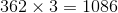### All SAT Math Resources